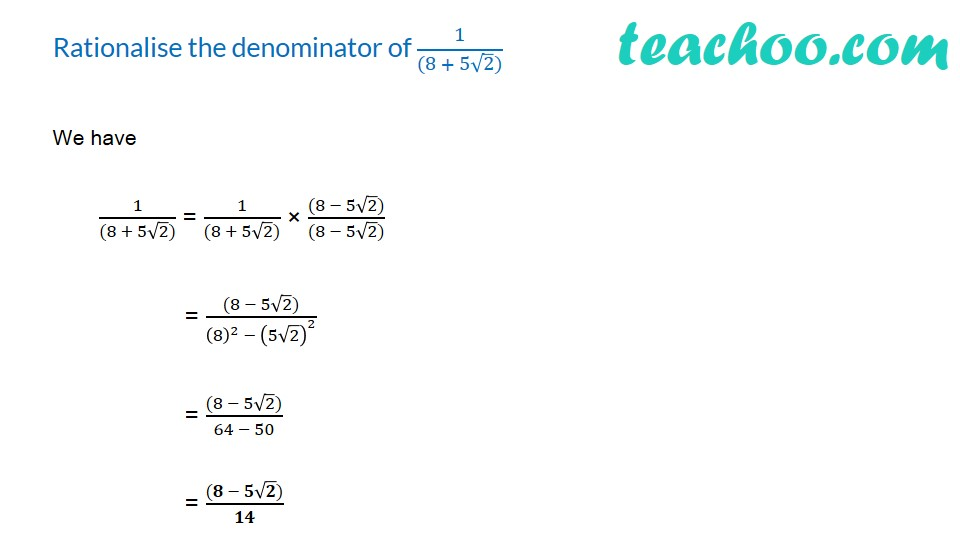Rationalising

Chapter 1 Class 9 Number Systems
Concept wiseIntroducing your new favourite teacher - Teachoo Black, at only ₹83 per month

### Transcript

Rationalise the denominator of 1/((8 + 5√2)) We have 1/((8 + 5√2)) = 1/((8 + 5√2)) × ((8 − 5√2))/((8 − 5√2)) = ((8 − 5√2))/((8)^2 − (5√2)^2 ) = ((8 − 5√2))/(64 − 50) = ((𝟖 − 𝟓√𝟐))/𝟏𝟒